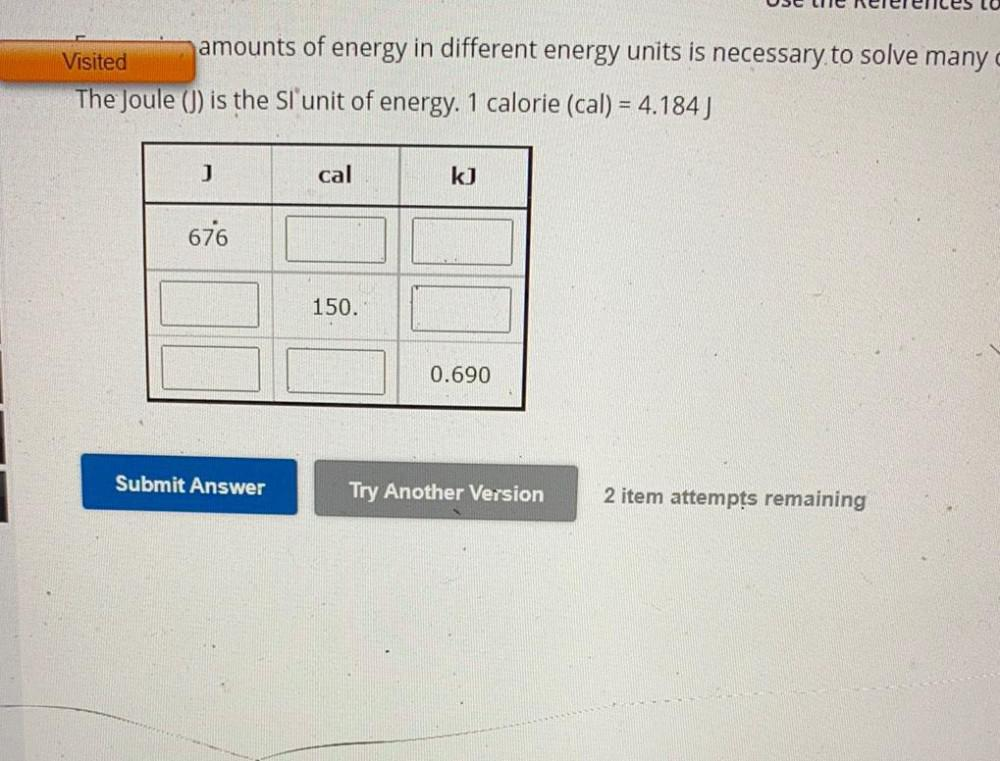Question:

# amounts of energy in different energy units is necessary to solve many Visited The Joule (J) is the SI unit of energy. 1 calorieamounts of energy in different energy units is necessary to solve many Visited The Joule (J) is the SI unit of energy. 1 calorie (cal) = 4.184) J cal k] 676 150. 0.690 Submit Answer Try Another Version 2 item attempts remaining